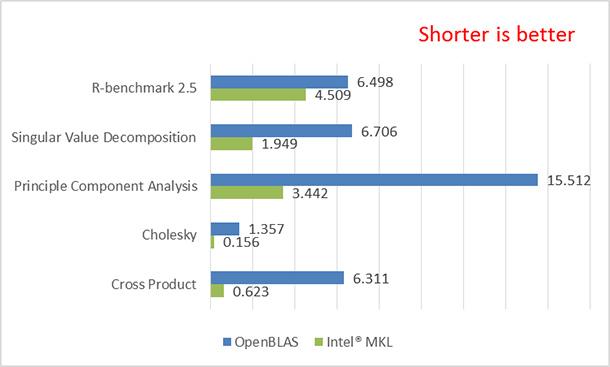# Performance Comparison of OpenBLAS* and Intel® oneAPI Math Kernel Library (oneMKL) in R

ID 672758
Updated 2/28/2022
Version Latest
PublicBy

## Introduction

Today, scientific and business industries collect large amounts of data, analyze them, and make decisions based on the outcome of the analysis. They employ data visualization techniques and predictive analytics to predict future probabilities and trends. R is a programming language for computational statistics, data visualization, and predictive analytics . Since data visualization and predictive analytics are compute intensive, it’s important to find ways to speed up the computing process in order to allow faster business and scientific decision making. This paper compares the performance of Basic Linear Algebra Subprograms (BLAS) , libraries OpenBLAS , and Intel® oneAPI Math Kernel Library (oneMKL) .

## Performance Test Procedure

Performance is measured based on how long (in seconds) it takes to run the tests. To compare the performance of the libraries, we performed the tests on a system equipped with the Intel® Xeon® processor E5-2697 v4. We first loaded the OpenBLAS and ran the tests. Next, we loaded the Revolution R and oneMKL and then reran the tests. We created simple tests to measure how long it takes to perform certain R functions. For example, to measure the performance of the cross product and Cholesky  function of a matrix, we followed these steps:

1. Create a matrix A.
2. Measure the time of the cross product of A using the following command:
system.time (crossprod(A))
3. Measure the time of the cholesky of A using the following command:
system.time (chol(A))

The following tests were performed:

• The cross product of a matrix (R function crossprod)
• The Cholesky decomposition of a matrix (R function chol)
• Singular value decomposition (R function svd) 
• Principal component analysis (R function prcomp) 
• R-benchmark v2.5 (this benchmark has a total of 15 tests.)

## Test Configurations

Hardware

• System: Preproduction
• Processor: Intel Xeon processor E5-2697 v4 @2.3 GHz
• Cores: 18
• Memory: 128 GB DDR4

Software

• RedHat Enterprise Linux* 7.0
• R 3.2.2
• Revolution R* 3.2.2
• OpenBLAS 0.2.14
• oneMKL (from revomath-3.2.2)

Note: Revolution R  was used here as a mean to test R functions with oneMKL since it is, by default, linked to oneMKL.

## Test ResultsFigure 1: The elapsed time of the tests OpenBLAS* versus Intel® oneAPI Math Kernel Library (oneMKL).

Figure 1 only shows the total elapsed time of the R-benchmark-25  test. The results are sorted in ascending order of oneMKL performance improvement.Figure 2: The R-benchmark-25 detail results of OpenBLAS* versus Intel® oneAPI Math Kernel Library (oneMKL).

Figure 2 shows the individual results of the R-benchmark v. 2.5. The results are sorted in ascending order of oneMKL performance improvement. oneMKL outperformed OpenBLAS on almost all the tests except the final test, Escoufier’s method on a 45x45 matrix. More information about Eigenvalues, Fibonacci, Hilbert, and Toeplitz can be found at , , , and  in the reference section, respectively.

Benefits of Using Intel® oneMKL

The results of figures 1 and 2 show that using oneMKL on systems equipped with Intel® Xeon® processors E5-2697 v4 product family helps speed up the R functions such as cross product, Cholesky decomposition, singular value decomposition (SVD), and so on as compared to using OpenBLAS. These functions are important in teaching machine learning (ML) methods and modern data analysis. oneMKL helps improve the performance of those functions by taking advantage of special features in Intel Xeon processor E5 v4 called Intel® Advanced Vector Extensions 2 (Intel® AVX2) that boosts the performance of matrix manipulation. The Intel Xeon processor E5 v4 implemented a hardware feature called fused multiply-add (FMA)  that greatly speed ups the multiply-add operation that is used extensively in matrix manipulation. For more information about FMA, go to www.software.intel.com. As new Intel® Xeon® processors launch with more improved architecture, newer version of oneMKL will make use of new features to optimize the above functions even more without the need for user intervention.

Conclusion

R plays an important role in analyzing data. Speeding up R will help improve performance of data analysis tools. Since data analysis tools heavily involve matrix computation, in general, oneMKL will help speed up these tools because oneMKL takes advantage of special features like Intel AVX2 that greatly speed up matrix calculation. With oneMKL, you don’t need to modify R source code. Just make sure to link the R compiler to the latest version of oneMKL to take advantage of new features in new Intel Xeon processors.

This is the first article in the ML series. Upcoming articles will discuss how oneMKL helps speed up not only at the functional level but also at the application level. We will also have articles discussing how to use and optimize ML applications using Python*.

References

 What is R?

 Basic Linear Algebra Subprograms - Wikipedia, the free encyclopedia

 Cholesky decomposition – Wikipedia, the free encyclopedia

 Singular value decomposition - Wikipedia, the free encyclopedia

 Principle component analysis - Wikipedia, the free encyclopedia

 Revolution R

 Eigenvalue – from Wolfram MathWorld

 Hilbert matrix - Wikipedia, the free encyclopedia

 Toeplitz matrix - Wikipedia, the free encyclopedia

 Multiply–accumulate operation - Fused multiply.E2.80.93add - Wikipedia, the free encyclopedia